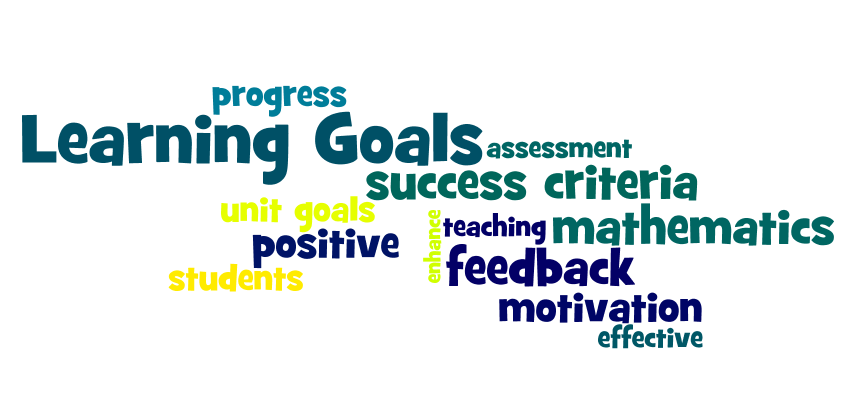# Learning GoalsLearning Goals in Mathematics

This year we are placing increased importance on Learning Goals in all of our classrooms from prep to year 6.

Learning Goals are important as they provide students with a map of their learning.  They make learning visible for students by clearly stating the knowledge and processes that are essential to a student’s success in the unit.

Our focus this year is on developing Learning Goals in mathematics.  Teachers work collaboratively to develop a specific set of unit Learning Goals for each mathematics unit as a part of our Reflective Teaching and Learning Cycle.

These Learning Goals are unpacked with students at the start of each unit and are referred to throughout the unit to ensure students understand where they are headed and how this activity fits into these unit goals.

Each teacher will enhance student’s engagement with Learning Goals in mathematics throughout the year by:

Displaying the learning goals and their success criteria

Planning activities that support the learning goals

Making explicit links between activities / lessons and learning goals

YEAR LEVEL Term 3, 2015 Learning Goals Join 2 groups together Identify how many all togetherRepresents addition stories using pictures and concrete materialsCreate an addition story Students can count in 1’s to 120.Students can skip count in 2’s to 50.Students can count 5’s to 120.Students can count 10’s to 150.Students can count on 0’s, 1’s and 2’s and count back 0’s, 1’s and 2’s.Students can count backwards from 20.Students can identify and write numbers 10 more and 10 less.Students can use a number board to identify missing numbers.Students can locate and order numbers to 50 (eg number lines)Students can represent 2 digit numbers in a variety of ways (for above 20 –  100 number of the day)Students can represent addition problems as part/part/whole.Students can represent simple subtraction problems using concrete materials and part/part/whole, fact families.Students can describe a half as 2 equal parts.Students can use coins to show value and coin combinations.Students can name the months of the year.Students can identify time using minutes, hours, o’clock, ½ past.Students can identify duration of time using minutes, hours and days.Students can use informal units to measure capacity. Students can identify situations of chance using appropriate language eg. will, won’t and may happen. Students will recognise, represent and use standard partitioning of 3 digit numbers.Students will count by 1s, 2s, 5s, 10s forwards and backwards to 1000. Students can use a number line and the part, part whole model to calculate change  Students can represent fractions in a variety e.g. collections, word forms, pictures and symbolsStudents can represent multiplication and division facts using arrays, groups and part, part wholes Students will be able to: order numbers up to two decimal places on a number line.represent decimals in word and numeral form.use a strategy to calculate cost and change to the nearest 5c.tell time for am/pm to the hour, half hour, quarter past/to.tell time for am/pm to the hour, half hour, quarter past/to and 5 minutes intervals and to the minute.use operations to solve problems involving duration and conversion of time.recall the 7 and 9 timetables and division facts. Students will be able to read, write, compare, order and represent unit fractions and decimals to hths and beyond. Students will be able to identify and use efficient mental and written to solve problems.Students will be able to write equivalent number sentences involving all operations to find unknown quantities.Students will be able to use extended multiplication and division facts.Students will be able to represent probabilities of outcomes ranging from 0 – 1.Students will be able to use appropriate units of measurement – length, area, volume, capacity.Students will be able identify, compare, estimate, measure and construct angles. Students will connect three-dimensional objects with their nets and other two-dimensional representations. Students will describe translations, reflections and rotations of two-dimensional shapes and identify line and rotational symmetries. ​Children can identify and apply angle relationships for angles on a straight line, vertically opposite angles and angles at a pointChildren can identify and use order of operations to solve problems Children will be able to position positive and negative numbers on a number lineChildren will be able to interpret positive and negative numbers in a real life situation Children can identify number properties and patterns and solve problems with them